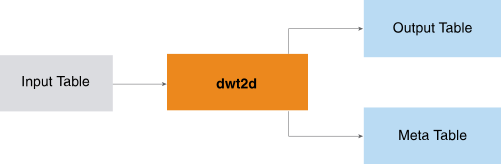# 1.1 - 8.10 - DWT2D (ML Engine) - Teradata Vantage

## Teradata Vantage™ - Machine Learning Engine Analytic Function Reference

Product
Release Number
1.1
8.10
Published
October 2019
Content Type
Programming Reference
Publication ID
B700-4003-079K
Language
English (United States)

The DWT2D function implements the Mallat algorithm (an iterate algorithm in the Discrete Wavelet Transform field) on 2-dimensional matrixes and applies wavelet transform on multiple sequences simultaneously.

The input is a set of sequences. Typically, each sequence is a matrix that contains a position in 2-dimensional space (y and x indexes or coordinates) and its corresponding values. You specify the wavelet name or wavelet filter table, transform level, and (optionally) extension mode. The function returns the transformed sequences in Hilbert space with the corresponding component identifiers and indices. (The transformation is also called the decomposition.)The wavelet filter table does not appear in the preceding diagram because it is seldom used.

This is a typical DWT2D use case:

1. Apply DWT2D to the original data to create the approximate coefficients of the matrixes and the corresponding metadata.
2. If desired, filter the coefficients by methods appropriate for the objects (for example, minimum threshold or top n coefficients).
3. From the approximate or filtered coefficients, reconstruct the matrixes and compare them with their original counterparts.